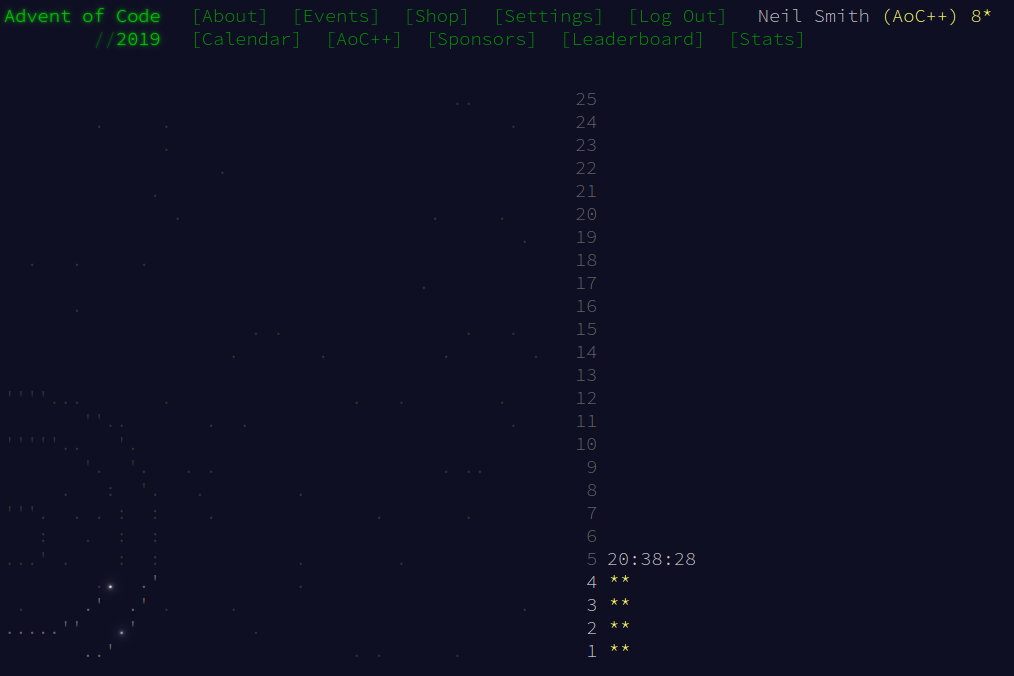December 4, 2019

# Advent of Code 2019 day 4

Disarmingly straightforward.Day 4 was… disarmingly straightforward. I thought I'd try with the most obvious approach, on the assumption that it would be too slow, but at least I'd have some parts for a better approach. Turns out, obvious was good enough.

The puzzle, basically, is to enumerate all six-digit numbers and reject those that fail a couple of tests. There are 106 such numbers, which isn't a lot for a standard PC. The constraint on the first digit (1–6 inclusive) reduces that range by about two-thirds, and the monotonically-non-decreasing constraint will about halve the number of candidates checked. That leaves about 3 × 105 numbers, which is fine.

The three tests are:

1. The digits are monotonically non-decreasing (each digit is at least as large as the digit to its left).
2. Two adjacent digits are the same.
3. When evaluated as a number, that number is within the range given.

Elegantly, Eric (the puzzle setter) avoided the question of whether the range limits were inclusive or exclusive by having those limits be invalid passwords by the non-decreasing constraint.

## Part 1

I decided to keep the candidate passwords as a 6-tuple of digits, rather than a number. It would make the first two tests simple, and converting a tuple of digits to a number is simple enough. I can generate all the candidates with a list comprehension, and chain the generators together to ensure the non-decreasing constraint.

candidates = [ (d1, d2, d3, d4, d5, d6)
| d1 <- [1..6]
, d2 <- [d1..9]
, d3 <- [d2..9]
, d4 <- [d3..9]
, d5 <- [d4..9]
, d6 <- [d5..9]
]


I then wrote the other two constraints as predicates: digit tuples that met the constraint would give a value of True. (&& is logical and; || is logical or.)

inRange digits = n >= lowerLimit && n <= upperLimit
where n = numify digits

numify :: (Int, Int, Int, Int, Int, Int) -> Int
numify (d1, d2, d3, d4, d5, d6) =
d1 * 10^5 + d2 * 10^4 + d3 * 10^3 + d4 * 10^2 + d5 * 10 + d6

adjacentSame (d1, d2, d3, d4, d5, d6) =
d1 == d2 || d2 == d3 || d3 == d4 || d4 == d5 || d5 == d6


All that remains is to filter the candidates by these predicates and count the number of passwords that remain.

part1 = length $filter inRange$ filter adjacentSame candidates


…and it ran very quickly. Result!

## Part 2

This altered the "adjacent digits" constraint, so I just wrote another predicate that encoded it.

isolatedAdjacentSame (d1, d2, d3, d4, d5, d6) =
(d1 == d2 && d2 /= d3)
|| (d1 /= d2 && d2 == d3 && d3 /= d4)
|| (d2 /= d3 && d3 == d4 && d4 /= d5)
|| (d3 /= d4 && d4 == d5 && d5 /= d6)
|| (d4 /= d5 && d5 == d6)


i.e. the second and third digits form an isolated pair if they're the same, and different from the first and fourth digits.

And that gives a slightly different pipeline:

part2 = length $filter inRange$ filter isolatedAdjacentSame candidates


## A variation

A slightly different approach is to apply the digit-based tests, then convert the digit-tuples into numbers, then test the numeric range constraints. That gives the pipeline:

part1a = length $filter (>= lowerLimit)$ filter (<= upperLimit)
$map numify$ filter adjacentSame candidates


As far as I can tell, it runs us just about the same amount of time and space as the original method. I'm not sure if it's any clearer to read.

## Code

The code is on Github., but Frerich's code uses the same approach and is more elegant than mine.